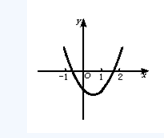2018年成人高考高起点文数考试章节难点解析（2）

难点4 三个“二次”及关系

●难点磁场

●案例探究

[例1]已知二次函数f(x)=ax2+bx+c和一次函数g(x)=-bx，其中a、b、c满足a>b>c,a+b+c=0,(a,b,c∈R).

(1)求证：两函数的图象交于不同的两点A、B;

(2)求线段AB在x轴上的射影A1B1的长的取值范围.

(1)证明：由 消去y得ax2+2bx+c=0

Δ=4b2-4ac=4(-a-c)2-4ac=4(a2+ac+c2)=4[(a+ c2]

∵a+b+c=0,a>b>c,∴a>0,c<0

∴ c2>0,∴Δ>0,即两函数的图象交于不同的两点.

(2)解：设方程ax2+bx+c=0的两根为x1和x2,则x1+x2=- ,x1x2= .

|A1B1|2=(x1-x2)2=(x1+x2)2-4x1x2

∵a>b>c,a+b+c=0,a>0,c<0

∴a>-a-c>c,解得 ∈(-2,- )

∵ 的对称轴方程是 .

∈(-2,- )时，为减函数

∴|A1B1|2∈(3,12),故|A1B1|∈( ).

[例2]已知关于x的二次方程x2+2mx+2m+1=0.

(1)若方程有两根，其中一根在区间(-1，0)内，另一根在区间(1，2)内，求m的范围.

(2)若方程两根均在区间(0，1)内，求m的范围.∴ .

(2)据抛物线与x轴交点落在区间(0，1)内，列不等式组 (这里0<-m<1是因为对称轴x=-m应在区间(0，1)内通过)

●锦囊妙计

1.二次函数的基本性质

(1)二次函数的三种表示法：

y=ax2+bx+c;y=a(x-x1)(x-x2);y=a(x-x0)2+n.

(2)当a>0,f(x)在区间[p,q]上的最大值M，最小值m,令x0= (p+q).

2.二次方程f(x)=ax2+bx+c=0的实根分布及条件.

(1)方程f(x)=0的两根中一根比r大，另一根比r小 a·f(r)<0;

(2)二次方程f(x)=0的两根都大于r

(3)二次方程f(x)=0在区间(p,q)内有两根 (4)二次方程f(x)=0在区间(p,q)内只有一根 f(p)·f(q)<0,或f(p)=0(检验)或f(q)=0(检验)检验另一根若在(p,q)内成立.

(5)方程f(x)=0两根的一根大于p,另一根小于q(p

3.二次不等式转化策略

(1)二次不等式f(x)=ax2+bx+c≤0的解集是：(-∞,α )∪[β,+∞ a<0且f(α)=f(β)=0;

(2)当a>0时，f(α)

|β+ |;

(3)当a>0时，二次不等式f(x)>0在[p,q]恒成立 或 (4)f(x)>0恒成立

|
【上一篇文章】:2018年成人高考高起点文数考试章节难点解析（1）
【下一篇文章】:2018年成人高考高起点文数考试章节难点解析（3）